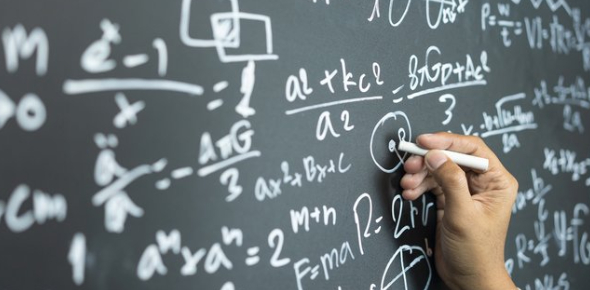# Tough Test On Mathematics: Quiz!

14 Questions | Total Attempts: 74Settings.

• 1.
What is the cardinality of the set {a, {a}, {a, {a}}}
• A.

3

• B.

4

• C.

Does not exist

• D.

0

• 2.
How many elements do the following settings have where a and b are distinct elements?  P({a, b, {a, b}})
• A.

8

• B.

16

• C.

4

• D.

32

• 3.
The sets A and B if A – B = {1, 5, 7, 8},  B – A = {2, 10} and A intersection B = {3, 6, 9}
• A.

A = {1, 3, 5, 6, 7, 8, 9}; B = {2, 3, 6, 9, 10}

• B.

A = {1, 2, 5, 6, 7, 8}; B = {2, 3, 9}

• C.

A = {3, 5, 6, 8, 9}; B = {2, 6, 9, 10}

• D.

A = {1, 2, 3, 4, 5, 6, 8, 9 10}; B = {2, 3, 6, 9}

• 4.
What can you say about the sets A and B if we know that A – B = A
• A.

A and B are disjoint

• B.

A is empty set

• C.

A intersection B is non-empty

• D.

A union B is equal to A intersection B

• 5.
The symmetric difference of {1, 3, 5} and {1, 2, 3}
• A.

{2, 5}

• B.

{1, 2, 5}

• C.

{1, 3, 4}

• D.

{1, 3}

• 6.
What can you say about the sets A and B if A symmetric difference B = A
• A.

B is empty set

• B.

A is empty set

• C.

A = B

• D.

A - B = B

• 7.
Suppose the universal set is U = {1, 2, 3, 4, 5, 6, 7, 8, 9, 10} express the following set with bit strings where the ith bit in the string is 1 if i is the set and 0 otherwise {2, 3, 4, 7, 8, 9}
• A.

0111001110

• B.

1111001010

• C.

0001110011

• D.

1011000110

• 8.
Let R be a binary relation on A such that (a, b) belongs to R if book ‘a’ costs more and contains fewer pages than book ‘b’.  What can you say about the relation?
• A.

Not reflexive, not symmetric, anti-symmetric and transitive

• B.

Reflexive, not symmetric, anti-symmetric and transitive

• C.

Not reflexive, not symmetric, not anti-symmetric, not transitive

• D.

Reflexive, not symmetric, not anti-symmetric and transitive

• 9.
Let R be a binary relation on the set of all positive integers such that R = {(a, b)/ a – b is an odd positive integer}
• A.

Not reflexive, not symmetric, anti-symmetric and transitive

• B.

Not reflexive, symmetric, anti-symmetric and not transitive

• C.

Reflexive, not symmetric, anti-symmetric and transitive

• D.

Reflexive, symmetric, not anti-symmetric and not transitive

• 10.
If R = {(a, b)/ a = b2} where a, b are positive integers, then R
• A.

Neither equivalence nor partial ordering relation

• B.

Reflexive and symmetric but not transitive

• C.

Reflexive and anti-symmetric but not transitive

• D.

Not reflexive, but anti-symmetric and transitive

• 11.
Let R on the set of all strings 0’s and 1’s such that R = {(a, b)/ a and b are strings that have same number of 0’s}
• A.

R is an equivalence but not anti-symmetric

• B.

R is a partial ordering relation but not symmetric

• C.

R is both equivalence and partial ordering relation

• D.

R is neither equivalence nor partial ordering relaiton

• 12.
If |A union B| = 12, A is subset of B and |A| = 3, then |B| is
• A.

12

• B.

9

• C.

Less than or equal to 9

• D.

None

• 13.
{[(A union B) intersection A] union B} intersection A is
• A.

A

• B.

B

• C.

A union B

• D.

A intersection B

• 14.
A relation S is defined as aSb if a2 + b2 = 4, find domain of S and range of S, where S is a relation from Z to Z.
• A.

D(S) = R(S) = {0, 2, -2}

• B.

D(S) = {2}; R(S) = {0}

• C.

D(S) = R(S) = {2}

• D.

D(S) = {0, 2}; R(S) = {0, 2, -2}

Related TopicsBack to top